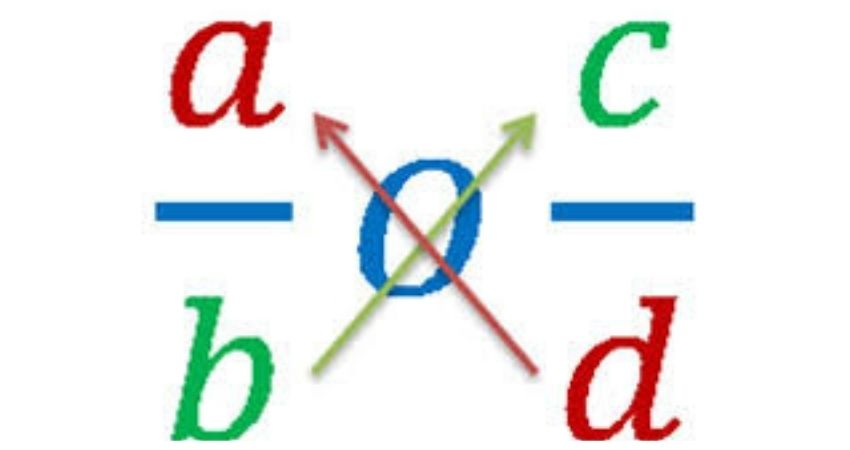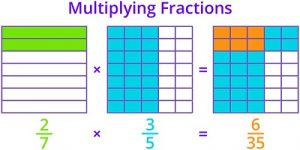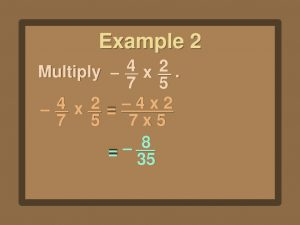June 27, 2022

# What Are Fractions And How To Multiply ThemIn mathematics, we have whole numbers like 1, 2, 3, 4, etc., and then there are also numbers that come in between these numbers like 1.20, 2.25, 3.25, 4.50, etc. We can write these numbers in two ways, one by writing the whole part first, followed by the fractional part and separated by a dot, and second by denoting as fractions. Fractions mean a part of the whole. They are written as two numbers, one on top of the other and separated by a line or a fraction bar. The number on top is called the numerator, and below one is called the denominator. Examples of fractions are 1/2, 3/4, 2/5, 5/4, etc.

If we have a number in decimal form, let us see how to convert it into a fraction. If we want to convert 2.5 to a fraction, then we first write down the number, divided by 1 (2.5/1), now start with multiplying numerator and denominator by 10 for each of the numbers appearing after the decimal notation. In this case, it will be 10 as there is one digit after the decimal.Hence, 2.5/1x 10/10= 25/10

As we have seen now, how decimals can be converted into fractions, let us now proceed to see how multiplying fractions works, which is very easy. When we multiply two fractions, we multiply the numerators and denominators together to get the respective product numerator and denominator.

Algebraically it can be understood as: a/bxc/d=a X c/b X d

Let us consider an example, multiply 4/5 and 2/7.

To solve this multiply together the numerators and the denominators

(4 x 2)/(5 x 7) = 8/35Multiplying fractions can be made easier by canceling out equal factors if present in the numerator and the denominator. This will make the numbers smaller, and the multiplication process becomes easier then.

If the fractions need to be divided, cross-multiplication can be used to solve the division. To cross-multiply two fractions, follow the below steps:

1. The numerator of the first fraction is to be multiplied by the denominator of the second fraction
2. The numerator of the second fraction is to be multiplied by the denominator of the first fraction

For example, if we have to divide these two fractions: 4/7 and 5/6

When we cross-multiply, we get these two numbers: 4 x 6 = 24 and 7 x 5 = 35

Cross-multiplication enables comparing the fractions and finding out the greatest. It is important to keep in mind to start with the numerator of the first fraction first.When something is divided into parts, fractions come into the picture, and along with it, then rises the need to add or multiply them. While multiplying fractions, you may have observed one thing that multiplication does not always result in a bigger fraction in fact, many times, the product is a smaller fraction; this is something different from the multiplication of positive whole numbers, where they result in bigger numbers.

Fractions are an important concept in mathematics, and they find their utility in everyday life too. Hence it becomes important to have an in-depth understanding of addition, subtraction, multiplication as well as the division of fractions. This will help the students in understanding the further higher mathematical concepts too. Math worksheets for fractions can be practiced by the students, which will help them in exposing their brains to a variety of problems. Many websites these days provide easily downloadable worksheets, and Cuemath is one of them. They have interactive and also visually appealing worksheets so as to keep the students engaged throughout.

Also check some other education guides and information.